0

求助 Excel VBA 搜尋關鍵字後貼到其他的tab的寫法

1 則留言

0

cnchern0614, 你好

RoT 您好

1. VBA 根據縣市分類北中南三區
2. VBA 自動開新tab，並且各自命名"北"/"中"/"南"
3. VBA 自動將北中南區各縣市內容直接貼到各自"北"/"中"/"南"三個不同的tab中。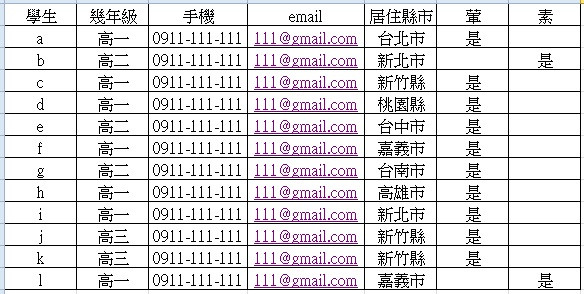RoT iT邦新手 5 級 ‧ 2020-11-16 23:10:40 檢舉

cnchern0614, 你好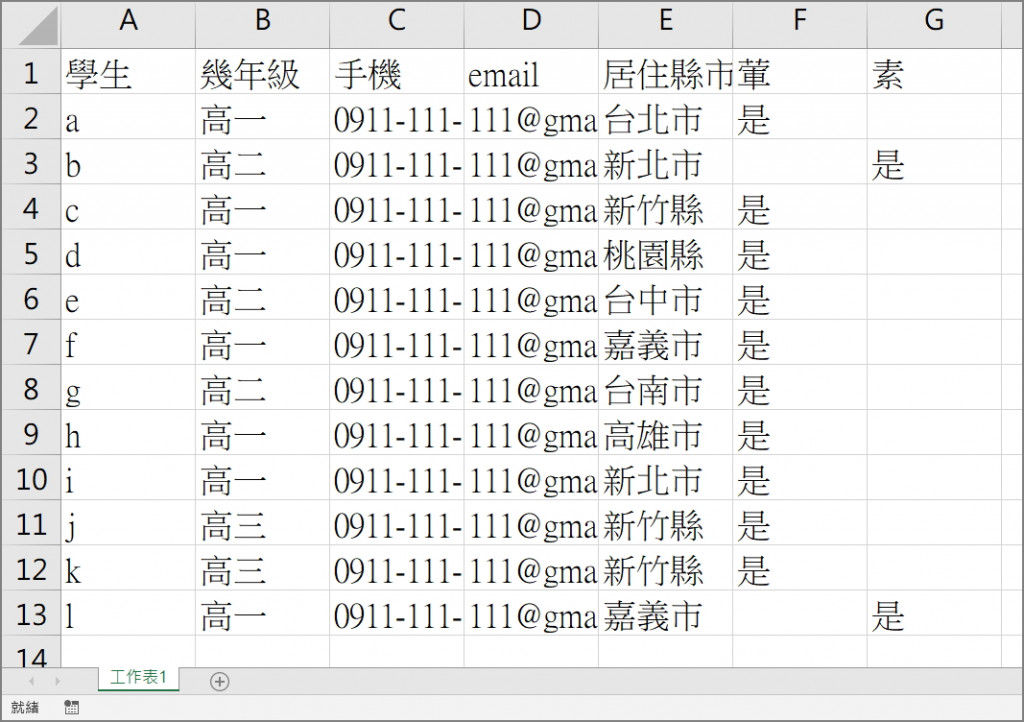Sub Sample()

Worksheets("工作表1").Activate
Worksheets("工作表1").Range("A1:G13").AutoFilter Field:=5, Criteria1:="=台北市"
Worksheets("工作表1").Range("A1:G2").Select
Selection.Copy
Worksheets("北").Range("A1").PasteSpecial
End Sub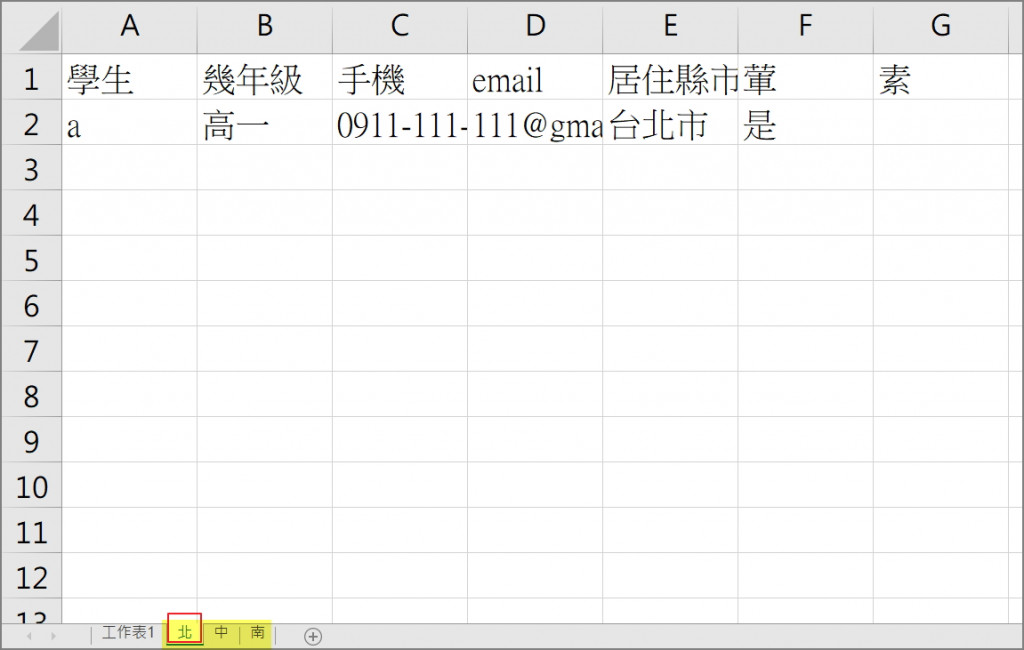Rot 您好

Sub Sample()

Worksheets("工作表1").Activate
Worksheets("工作表1").Range("A1:G13").AutoFilter Field:=5, Criteria1:="=台北市"
Worksheets("工作表1").Range("A1:G13").Select
Selection.Copy
Worksheets("北").Range("A1").PasteSpecial

Worksheets("工作表1").Activate
Worksheets("工作表1").Range("A1:G13").AutoFilter Field:=5, Criteria1:="=新北市"
Worksheets("工作表1").Range("A1:G13").Select
Selection.Copy
Worksheets("北").Range("A1").PasteSpecial

Worksheets("工作表1").Activate
Worksheets("工作表1").Range("A1:G13").AutoFilter Field:=5, Criteria1:="=桃園縣"
Worksheets("工作表1").Range("A1:G13").Select
Selection.Copy
Worksheets("北").Range("A1").PasteSpecial

Worksheets("工作表1").Activate
Worksheets("工作表1").Range("A1:G13").AutoFilter Field:=5, Criteria1:="=新竹縣"
Worksheets("工作表1").Range("A1:G13").Select
Selection.Copy
Worksheets("北").Range("A1").PasteSpecial

Worksheets("工作表1").Range("A1:G13").AutoFilter Field:=5, Criteria1:="=台中市"
Worksheets("工作表1").Range("A1:G13").Select
Selection.Copy
Worksheets("中").Range("A1").PasteSpecial

Worksheets("工作表1").Range("A1:G13").AutoFilter Field:=5, Criteria1:="=嘉義市"
Worksheets("工作表1").Range("A1:G13").Select
Selection.Copy
Worksheets("南").Range("A1").PasteSpecial

Worksheets("工作表1").Range("A1:G13").AutoFilter Field:=5, Criteria1:="=台南市"
Worksheets("工作表1").Range("A1:G13").Select
Selection.Copy
Worksheets("南").Range("A1").PasteSpecial

Worksheets("工作表1").Range("A1:G13").AutoFilter Field:=5, Criteria1:="=高雄市"
Worksheets("工作表1").Range("A1:G13").Select
Selection.Copy
Worksheets("南").Range("A1").PasteSpecial

End Sub
RoT iT邦新手 5 級 ‧ 2020-11-17 09:36:36 檢舉

cnchern0614, 你好

Worksheets("工作表1").Activate
Worksheets("工作表1").Range("A1:G13").AutoFilter Field:=5, Criteria1:="=台北市"
Worksheets("工作表1").Range("A1:G13").Select
Selection.Copy
Worksheets("北").Range("A1").PasteSpecial

Worksheets("工作表1").Activate
Worksheets("工作表1").Range("A1:G13").AutoFilter Field:=5, Criteria1:="=新北市"
Worksheets("工作表1").Range("A1:G13").Select
Selection.Copy
Worksheets("北").Range("A1").PasteSpecial

1. 問題一
Worksheets("工作表1").Range("A1:G13").Select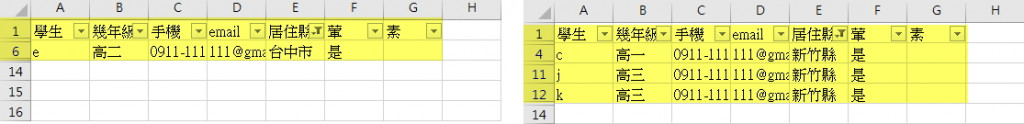1. 問題二
Worksheets("北").Range("A1").PasteSpecial
'這邊僅為 北 舉例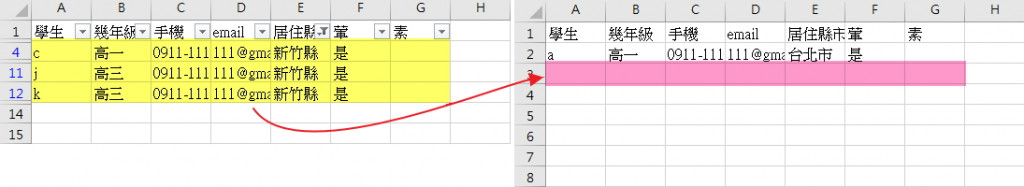RoT 您好

RoT iT邦新手 5 級 ‧ 2020-11-17 10:51:11 檢舉

cnchern0614, 你好

Sub Sample()

For i = 2 To 13
Worksheets("工作表1").Activate
Select Case Cells(i, 5).Value
Case Is = "台北市"
Worksheets("工作表1").Range("A" & i & ":G" & i).Select
Selection.Copy
Worksheets("北").Activate
Worksheets("北").Range("A" & Worksheets("北").Cells(Rows.Count, 1).End(xlUp).Row + 1).PasteSpecial
Case Is = "台南市"
Worksheets("工作表1").Range("A" & i & ":G" & i).Select
Selection.Copy
Worksheets("南").Activate
Worksheets("南").Range("A" & Worksheets("南").Cells(Rows.Count, 1).End(xlUp).Row + 1).PasteSpecial
End Select
Next i
End Sub

Rot 您好，抱歉現在才回覆，我剛剛有做些測試，已經能夠滿足我的使用，但是我有個地方想要再請教您一下

Case Is = "台北市 公館"
Worksheets("工作表1").Range("A" & i & ":G" & i).Select
Selection.Copy
Worksheets("台北市 公館").Activate
Worksheets("台北市 公館").Range("A" & Worksheets("台北市 公館").Cells(Rows.Count, 1).End(xlUp).Row + 1).PasteSpecial